# 欢迎关注WX公众号：【程序员管小亮】

## 专栏——Kaggle竞赛实战系列

### 一、介绍

MNIST 是计算机视觉领域的 hello world 数据集。自从1999年发布以来，这个经典的手写数字识别数据集就成为分类算法的基础，即使新的机器学习技术在不停地出现，但 MNIST 仍然是研究人员和学习者的可靠资源。

import pandas as pd
import numpy as np
import matplotlib.pyplot as plt
import matplotlib.image as mpimg
import seaborn as sns
%matplotlib inline

np.random.seed(2)

from sklearn.model_selection import train_test_split
from sklearn.metrics import confusion_matrix
import itertools

# 转换为独热编码
from keras.utils.np_utils import to_categorical
from keras.models import Sequential
from keras.layers import Dense, Dropout, Flatten, Conv2D, MaxPool2D, BatchNormalization
from keras.optimizers import RMSprop
from keras.preprocessing.image import ImageDataGenerator
from keras.callbacks import ReduceLROnPlateau

# 设置显示样式的参数
# http://seaborn.pydata.org/generated/seaborn.set.html
sns.set(style='white', context='notebook', palette='deep')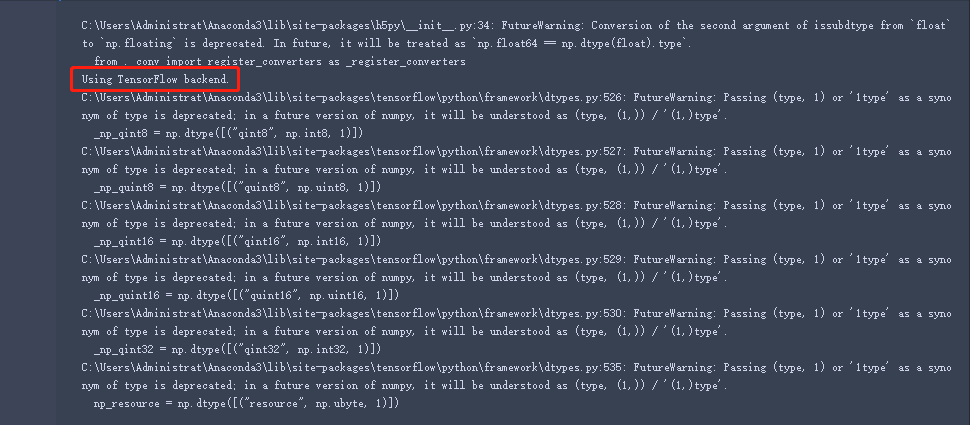import warnings
warnings.filterwarnings('ignore')


### 二、数据准备

#### 2.1、数据加载# 加载数据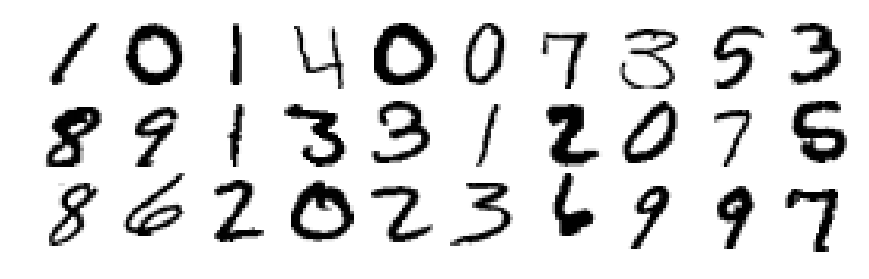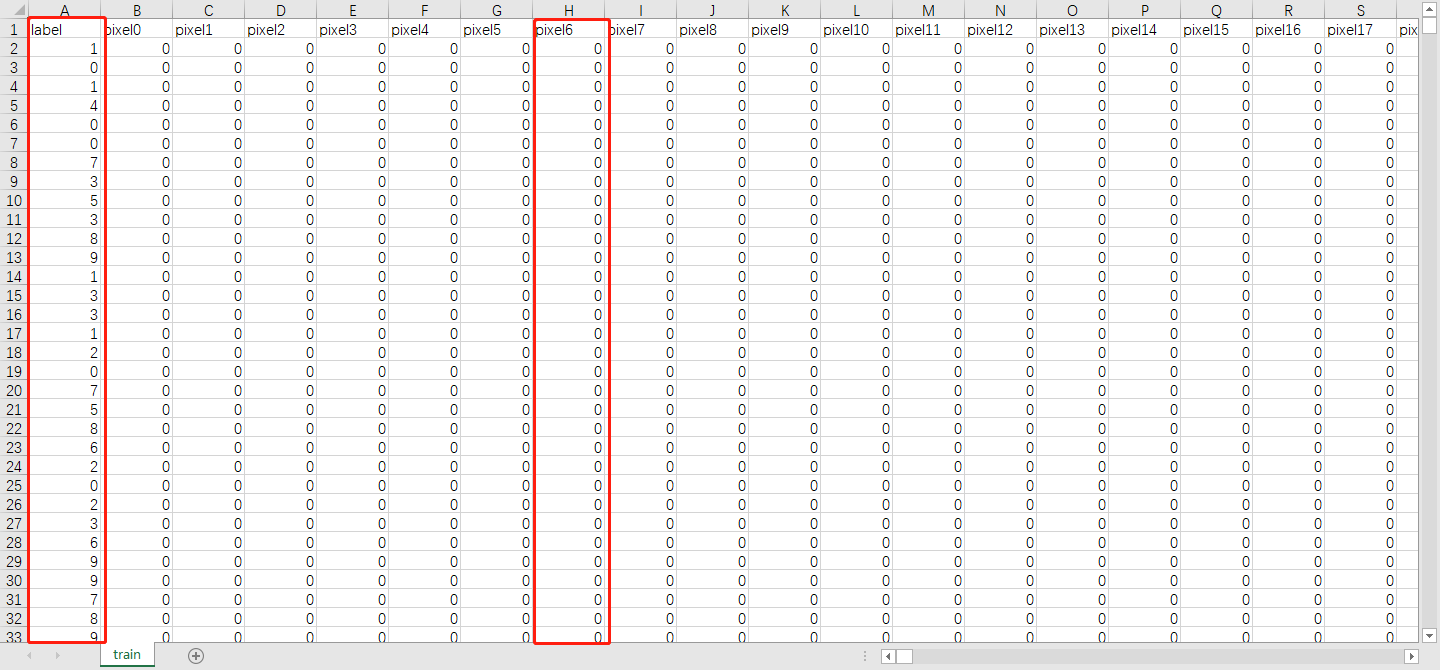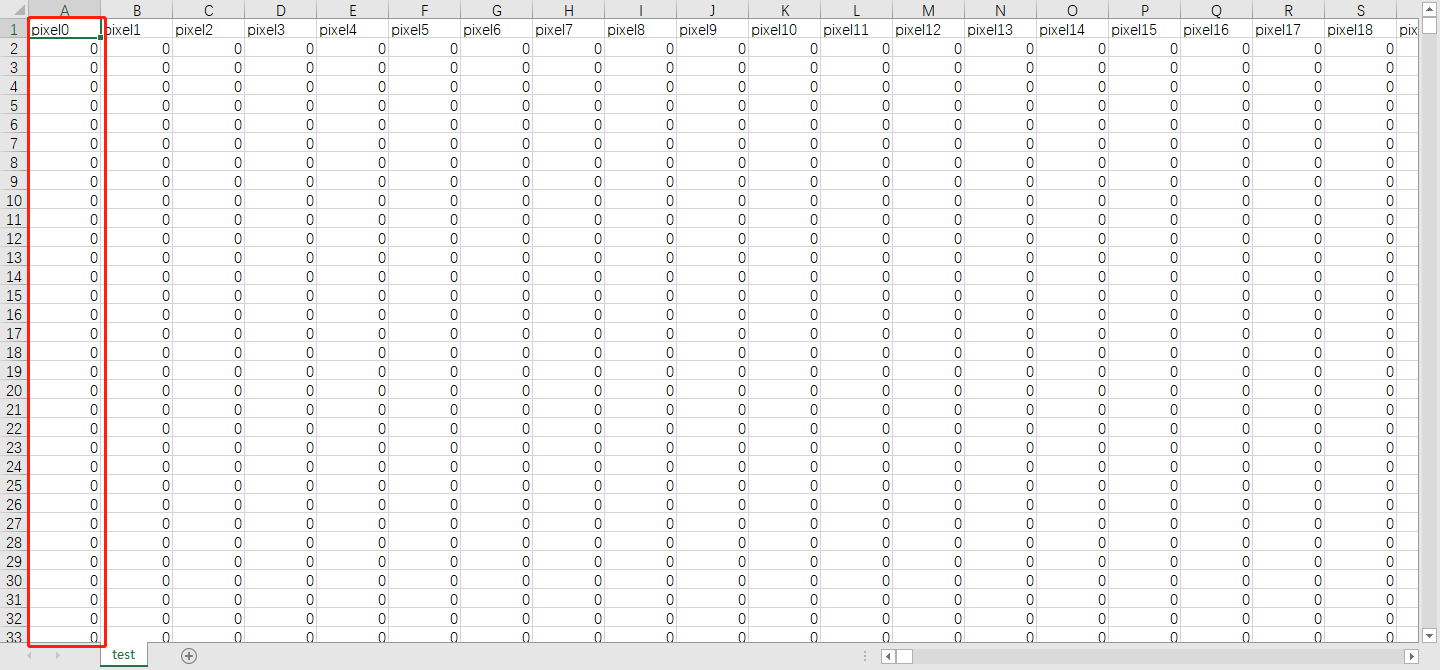#### 2.2、数据可视化

# 'label'
Y_train = train["label"]
print(Y_train.shape)

# 删除 'label' 列
X_train = train.drop(labels = ["label"],axis = 1)
print(X_train.shape)

# 释放一些空间
del train

# 使用条形图显示每个分类数据集合中的观测值
# https://seaborn.pydata.org/generated/seaborn.countplot.html?highlight=countplot
g = sns.countplot(Y_train)

# 对训练集中的元素计数
# https://pandas.pydata.org/pandas-docs/stable/reference/api/pandas.Series.value_counts.html
Y_train.value_counts()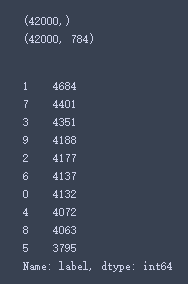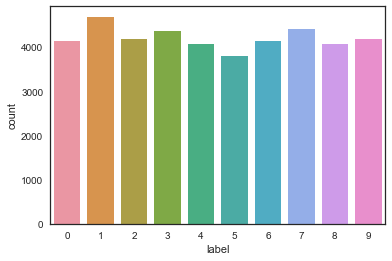#### 2.3、数据清洗

# 检查数据
X_train.isnull().any().describe()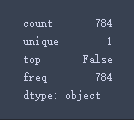X_train.isnull().any().describe() 将信息进行汇总：

• count 为总统计数；
• unique 为种类（由于该数据中没有空值，全为False，故只有1类）；
• top 为最多的种类；
• freq 为最多的种类出现的频次。
test.isnull().any().describe()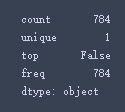#### 2.4、归一化

# 对数据进行归一化，到[0, 1]范围内，减小光照的影响，并可加速CNN收敛速度
X_train = X_train / 255.0
test = test / 255.0


#### 2.5、Reshape

# Reshape三维图像(height = 28px, width = 28px , canal = 1)
X_train = X_train.values.reshape(-1,28,28,1)
test = test.values.reshape(-1,28,28,1)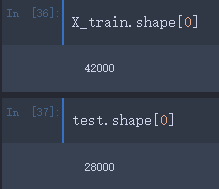#### 2.6、标签编码

# 将标签编码为一个独热向量 (例如: 2 -> [0,0,1,0,0,0,0,0,0,0])
Y_train = to_categorical(Y_train, num_classes = 10)


#### 2.7、分割交叉验证集

# 设置随机种子
random_seed = 2

# 分割出训练集和验证集
X_train, X_val, Y_train, Y_val = train_test_split(X_train, Y_train, test_size = 0.1, random_state=random_seed)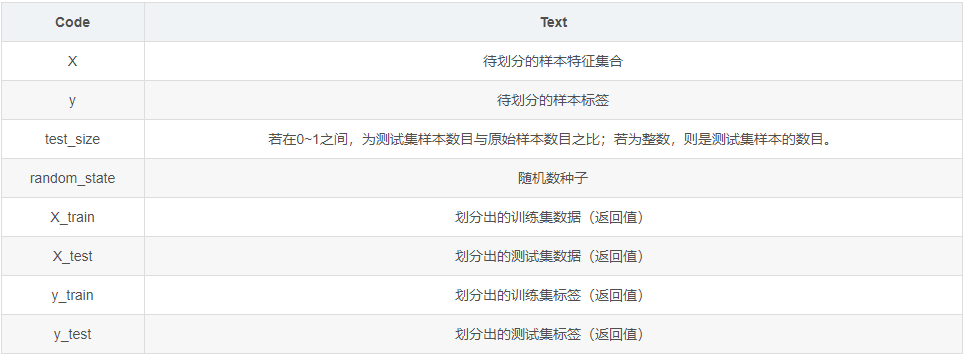print(X_train.shape)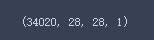# 一些例子
g = plt.imshow(X_train[:,:,0])g = plt.imshow(X_train[:,:,0])g = plt.imshow(X_train[:,:,0])### 三、卷积神经网络CNN

#### 3.1、定义网络模型

• 卷积（conv2d）层就像一组可学习的过滤器：前三个 conv2d 层设置32个过滤器，后三个层设置64个过滤器。

• 池化（maxpool2d）层是一个下采样滤波器：它着眼于2个相邻像素，并选择最大值。这些都是用来减少计算成本，并在一定程度上也减少了过拟合。

• 归一化层是一种正则化方法，可以加快收敛速度，控制并减少过拟合，同时还允许网络使用较大的学习率。

• Dropout 是一种正则化方法，其中某些层的部分节点被随机忽略（将其 wieghts 设置为零）。这将随机丢弃网络的一个属性，并强制网络以分布式方式学习特性。该方法还提高了泛化能力，减少了过拟合。

• relu 是线性整流函数，又称修正线性单元，也就是俗称的激活函数，公式是 max（0，x）relu 的主要作用就是向网络中添加非线性，故也称为非线性激活函数。

• Flatten 层用于将最终特征映射转换为一个一维向量，展开之后可以在某些 卷积/maxpool 层之后使用全连接层，它结合了以前卷积层提取的所有局部特征。

• 全连接（dense）层是用于实现分类，即人工神经网络分类器，在最后一层（Dense(10, activation='softmax')），网络输出每个类别的概率分布。

# 设置CNN模型
model = Sequential()

activation ='relu', input_shape = (28,28,1)))
activation ='relu'))

activation ='relu'))
activation ='relu'))

# 输出模型各层的参数状况
model.summary()#### 3.2、设置优化器和退火函数

• 损失函数用来衡量模型在带有已知标签的图像数据集上的性能有多差，它是目标标签和预测标签之间的错误率。使用最多的是交叉熵损失函数，即 categorical_crossentropy loss

• 优化器是最重要的功能，它将迭代地改进参数（filters kernel values, weights and bias of neurons ...），以最小化损失函数。

• 可以选择 rmsprop，它是一个非常有效的优化器，以一种非常简单的方式调整 adagrad 方法，试图降低其攻击性强、单调下降的学习率。
• 还可以使用 adam
• 也可以使用 sgd 优化器，但它比 rmsprop 慢。
• 度量函数 accuracy 用于评估模型的性能，不过仅用于评估。

# 用adam优化器和交叉熵损失进行编译

# 用sgd优化器
# model.compile(optimizer="sgd", loss="categorical_crossentropy", metrics=["accuracy"])

# 定义优化器
# optimizer = RMSprop(lr=0.001, rho=0.9, epsilon=1e-08, decay=0.0)
# 编译模型
# model.compile(optimizer = optimizer , loss = "categorical_crossentropy", metrics=["accuracy"])


# 设置一个学习率衰减
learning_rate_reduction = ReduceLROnPlateau(monitor='val_acc',
patience=3,
verbose=1,
factor=0.5,
min_lr=0.00001)

# 训练轮数，暂时设置为30，可以自己尝试调整
epochs = 30
# 批大小
batch_size = 86


#### 3.3、数据增强

# 增加数据以防止过拟合
datagen = ImageDataGenerator(
featurewise_center=False,  				# 在数据集上将输入平均值设置为0
samplewise_center=False,  				# 将每个样本的平均值设置为0
featurewise_std_normalization=False,    # 将输入除以数据集的std
samplewise_std_normalization=False,  	# 将每个输入除以它的std
zca_whitening=False,  					# 使用ZCA白化
rotation_range=10,  					# 在范围内随机旋转图像（0到180度）
zoom_range = 0.1, 						# 随机缩放图像
width_shift_range=0.1,  				# 水平随机移动图像（总宽度的一部分）
height_shift_range=0.1,  				# 垂直随机移动图像（总高度的一部分）
horizontal_flip=False,  				# 随机翻转图像
vertical_flip=False)  					# 随机翻转图像

datagen.fit(X_train)


• 训练图像随机旋转10度；
• 随机缩放10%一些训练图像；
• 将图像水平移动10%的宽度；
• 将图像垂直移动10%的高度；
• 没有应用垂直翻转或水平翻转，因为它可能导致错误分类对称数字，如6和9。

#### 3.4、拟合数据

# 拟合模型
history = model.fit_generator(datagen.flow(X_train,Y_train, batch_size=batch_size),
epochs = epochs, validation_data = (X_val,Y_val),
verbose = 2, steps_per_epoch=X_train.shape // batch_size,
callbacks=[learning_rate_reduction])### 四、评估模型

#### 4.1、训练和验证曲线

# 绘制训练和验证的损失和精度曲线
fig, ax = plt.subplots(2,1)
ax.plot(history.history['loss'], color='b', label="Training loss")
ax.plot(history.history['val_loss'], color='r', label="validation loss",axes =ax)

ax.plot(history.history['accuracy'], color='b', label="Training accuracy")
ax.plot(history.history['val_accuracy'], color='r',label="Validation accuracy")#### 4.2、混淆矩阵

# 看混淆矩阵
def plot_confusion_matrix(cm, classes,
normalize=False,
title='Confusion matrix',
cmap=plt.cm.Blues):
"""
此函数打印并绘制混淆矩阵
可以通过设置 “normalize=true” 应用归一化
"""
plt.imshow(cm, interpolation='nearest', cmap=cmap)
plt.title(title)
plt.colorbar()
tick_marks = np.arange(len(classes))
plt.xticks(tick_marks, classes, rotation=45)
plt.yticks(tick_marks, classes)

if normalize:
cm = cm.astype('float') / cm.sum(axis=1)[:, np.newaxis]

thresh = cm.max() / 2.
for i, j in itertools.product(range(cm.shape), range(cm.shape)):
plt.text(j, i, cm[i, j],
horizontalalignment="center",
color="white" if cm[i, j] > thresh else "black")

plt.tight_layout()
plt.ylabel('True label')
plt.xlabel('Predicted label')

# 从验证数据集中预测值
Y_pred = model.predict(X_val)
# 将预测类转换为一个独热向量
Y_pred_classes = np.argmax(Y_pred,axis = 1)
# 将验证观测转换为一个独热向量
Y_true = np.argmax(Y_val,axis = 1)
# 计算混淆矩阵
confusion_mtx = confusion_matrix(Y_true, Y_pred_classes)
# 绘制混淆矩阵
plot_confusion_matrix(confusion_mtx, classes = range(10))# 显示一些错误结果

# 错误是预测标签和真实标签之间的区别
errors = (Y_pred_classes - Y_true != 0)

Y_pred_classes_errors = Y_pred_classes[errors]
Y_pred_errors = Y_pred[errors]
Y_true_errors = Y_true[errors]
X_val_errors = X_val[errors]

def display_errors(errors_index,img_errors,pred_errors, obs_errors):
"""
此函数显示6个图像及其预测和实际标签
"""
n = 0
nrows = 2
ncols = 3
fig, ax = plt.subplots(nrows,ncols,sharex=True,sharey=True)
for row in range(nrows):
for col in range(ncols):
error = errors_index[n]
ax[row,col].imshow((img_errors[error]).reshape((28,28)))
ax[row,col].set_title("Predicted label :{}\nTrue label :{}".format(pred_errors[error],obs_errors[error]))
n += 1

# 错误预测数的概率
Y_pred_errors_prob = np.max(Y_pred_errors,axis = 1)

# 误差集中真值的预测概率
true_prob_errors = np.diagonal(np.take(Y_pred_errors, Y_true_errors, axis=1))

# 预测标签概率与真实标签概率之差
delta_pred_true_errors = Y_pred_errors_prob - true_prob_errors

# 对预测标签概率与真实标签概率之差的列表进行排序
sorted_dela_errors = np.argsort(delta_pred_true_errors)

# Top 6错误
most_important_errors = sorted_dela_errors[-6:]

# 展示Top 6错误
display_errors(most_important_errors, X_val_errors, Y_pred_classes_errors, Y_true_errors)#### 4.3、预测和提交

# 预测结果
results = model.predict(test)

# 选择最大概率的整数
results = np.argmax(results,axis = 1)
results = pd.Series(results,name="Label")

submission = pd.concat([pd.Series(range(1,28001),name = "ImageId"),results],axis = 1)

# 转换成CSV格式，不保留索引
submission.to_csv("cnn_mnist_datagen.csv",index=False)### 五、MNIST上的最佳模型

CNN的架构有很多选择，那么如何选择最好的一个呢？ 下面将会通过实验来进行测试。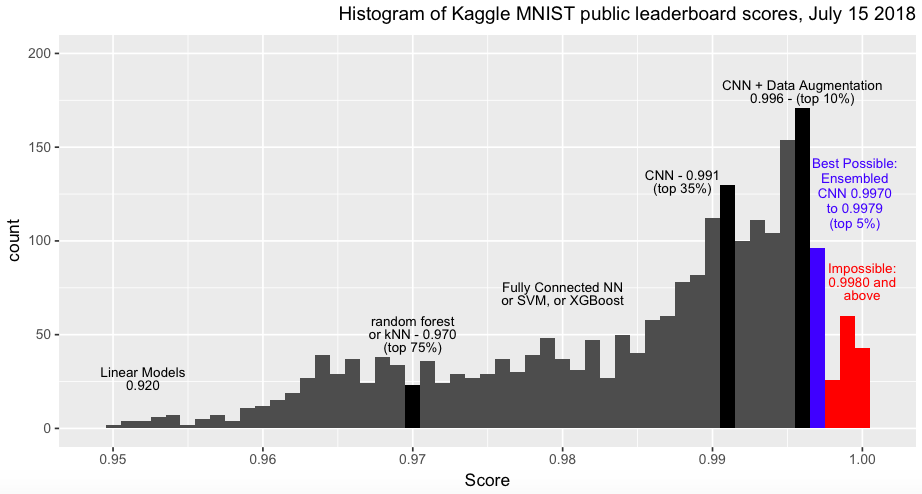• 92％：多项逻辑回归又名 softmax 回归是简单的尝试，得分为 92％
• 97％：非线性方法的得分为 97％，包括 kNN，随机森林等；
• 98％：非线性内核或全连接神经网络的 SVM 的得分为 98％，如果你调一调参，也许能到 99％
• 99％：卷积神经网络是图像分类的冠军，基本跑几轮就得分 99％

• 99.5％：一个设计好的CNN架构，然后添加了特殊功能，例如池化层，数据增强，Dropout，批归一化，学习率衰减，高级优化器等等，那么仅用20轮就可以突破 99.5％ 的里程碑！比如我们上面实验中使用的这个网络结构；
• 99.7%：要打破 99.7％，除了设计好的CNN架构，还要使用GPU，这样就不需要永远训练了，因为CPU跑的实在是太慢了！这种情况下，如果训练10次并进行10次结果提交，其中之一可能会超过 99.7％，因为每次训练CNN时，都会得到不同的结果；
• 99.8％-99.9％：要获得 99.8％ 或更高的分数，需要进行一次非常幸运的训练过程，或者需要使用 70,000 张图像的完整原始 MNIST 数据集进行训练，其中不公平地包含 Kaggle 的 test.csv 图像（这绝对是作弊），70,000 张图像的完整原始 MNIST 数据集的 地址在这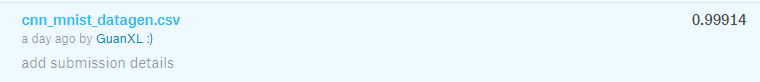• 100.0％：除了必须使用 70,000 张图像的完整原始MNIST数据集进行训练之外，还需要一个很好的算法支持，比如一个得分为 100％ 的Kaggle内核就是使用作弊算法 kNN

### 六、不应该被提交的结果c1=0; c2=0;
print("Classifying Kaggle's 'test.csv' using kNN k=1 and MNIST 70k images")
for i in range(0,28000): 		# 循环Kaggle测试集
for j in range(0,70000): 	# 循环MNIST数据集
# 如果数据相同，那么标签相同
if np.absolute(Kaggle_test_image[i,] - MNIST_image[j,]).sum()==0:
Kaggle_test_label[i] = MNIST_label[j]
if i%1000==0:
print("  %d images classified perfectly" % (i))
if j<60000:
c1 += 1
else:
c2 += 1
break
if c1+c2==28000:
print("  28000 images classified perfectly")
print("Kaggle's 28000 test images are fully contained within MNIST's 70000 dataset")
print("%d images are in MNIST-train's 60k and %d are in MNIST-test's 10k" % (c1,c2))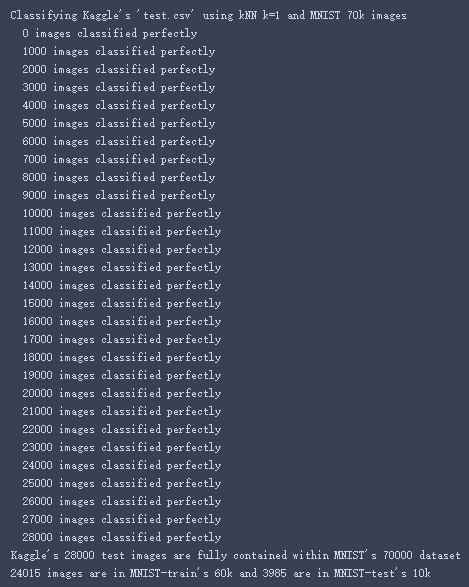# 输出找到的标签即可
results = pd.Series(Kaggle_test_label.reshape(28000,),name="Label")
submission = pd.concat([pd.Series(range(1,28001),name = "ImageId"),results],axis = 1)
submission.to_csv("Do_not_submit",index=False)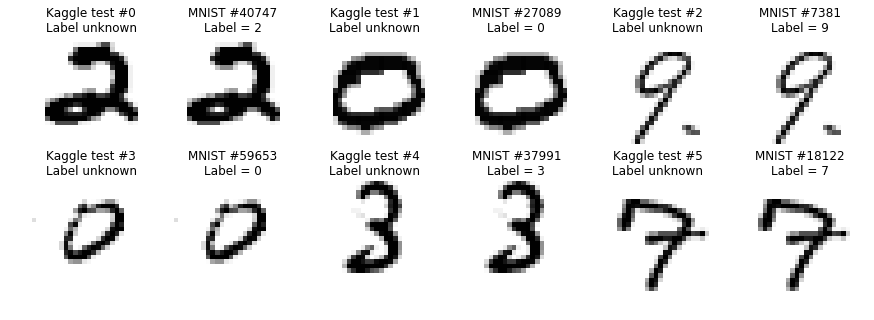### 七、MNIST上的最佳CNN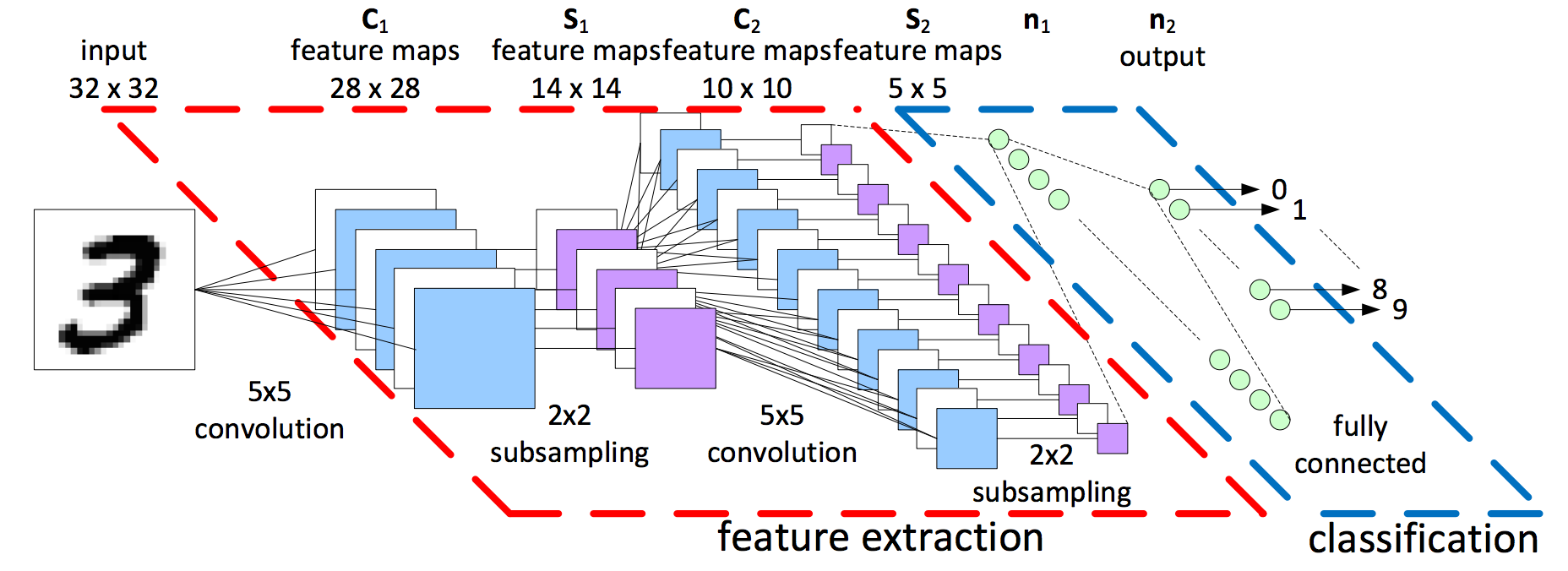https://www.kaggle.com/cdeotte/how-to-choose-cnn-architecture-mnist/notebook 中对【不同的卷积子空间对】、【特征图】、【全连接层】、【Dropout】、【归一化】、【数据增强】等等进行了分别的实验，发现了一下结构性能更高：

• 784(28 * 28) - [32C3-32C3-32C5S2](c=filter, s=stride) - [64C3-64C3-64C5S2] - 128 - 10
• 40% dropout，归一化，数据增强

### 八、初代网络 LeNet-5## 参考文章

• https://www.kaggle.com/kernels/svzip/notebook
• https://www.kaggle.com/cdeotte/how-to-choose-cnn-architecture-mnist/notebook
• https://www.kaggle.com/c/digit-recognizer/discussion/61480#latest-645703
• https://www.kaggle.com/cdeotte/mnist-perfect-100-using-knn/output#Accuracy=100%-using-kNN-k=1-and-MNIST-70k-images
12-0304-091033
07-115030
03-013万+
11-291844
01-061678
08-251141
07-06666
01-271万+
07-053183